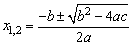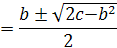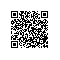# 从10W个数中随机抽走2个数，求出那两个数是多少

1、控制变量的个数使用（最多不允许超过5个）

2、不允许使用数组变量

3、不允许改变数组的值

现在的数组，指被抽走2个数且顺序被随机打乱了的数组。

   1: var n = 100* 1000;
   2: var arr = [];
   3:
   4: for (var i = 0; i < n ; i++) {
   5:     arr.push(i + 1);
   6: }
   7:
   8: var num1 = arr.splice(Math.floor(Math.random() * arr.length), 1);
   9: var num2 = arr.splice(Math.floor(Math.random() * arr.length), 1);
  10:
  11: document.write('抽掉数：<br/>第1个数是：' + num1 + '，第2个数是：' + num2 + '<br/><br/>');

   1: //打乱
   2: arr.sort(function() {return Math.random() > 0.5;});

   1: var n = 100* 1000;
   2: var arr = [];
   3:
   4: for (var i = 0; i < n ; i++) {
   5:     arr.push(i + 1);
   6: }
   7:
   8: var num1 = arr.splice(Math.floor(Math.random() * arr.length), 1);
   9: var num2 = arr.splice(Math.floor(Math.random() * arr.length), 1);
  10:
  11: document.write('抽掉数：<br/>第1个数是：' + num1 + '，第2个数是：' + num2 + '<br/><br/>');
  12:
  13: //打乱
  14: arr.sort(function() {return Math.random() > 0.9;});

x * x + y * y = c;

x + y = b              ①

x*x + y * y = c      ②

(b - y)*(b - y) + y * y = c

<==>

2y2 – 2by + b2 – c = 0

<==>

y2 – by + (b2 – c)/2= 0

b2 – 4ac  ==> b2- 2(b2 - c) ==> 2c – b2x + y =  原数组每一项之和 -  现在数组中每一项之和

x*x + y * y = 正常数组每一项的平方各 - 现在数组的每一项的平方各

1 + 2 + 3 + 4 + … + (n – 2 ) + (n - 1) + n 的求和通项公式为：n * (n + 1) / 2。

   1: //找出那两个数
   2: var t1 = 0,
   3:     t2 = 0,
   4:     t3 = 0,
   5:     len = arr.length;
   6:
   7: for (;t3 < n ; t3++) {
   8:
   9:     t2 += (t3 + 1) * (t3 + 1);
  10:
  11:     if (t3 < len) {
  12:         t1 += arr[t3];
  13:         t2 -= arr[t3] * arr[t3];
  14:     }
  15: }
  16:
  17: t3 = (n + 1) * n / 2 - t1;
  18:
  19: var x1 = (t3 - Math.sqrt(2 * t2 - t3 * t3)) / 2;
  20: var x2 = (t3 + Math.sqrt(2 * t2 - t3 * t3)) / 2;

   1: var n = 100* 1000;
   2: var arr = [];
   3:
   4: for (var i = 0; i < n ; i++) {
   5:     arr.push(i + 1);
   6: }
   7:
   8: var num1 = arr.splice(Math.floor(Math.random() * arr.length), 1);
   9: var num2 = arr.splice(Math.floor(Math.random() * arr.length), 1);
  10:
  11: document.write('抽掉数：<br/>第1个数是：' + num1 + '，第2个数是：' + num2 + '<br/><br/>');
  12:
  13: //打乱
  14: arr.sort(function() {return Math.random() > 0.9;});
  15:
  16:
  17: //找出那两个数
  18: var t1 = 0,
  19:     t2 = 0,
  20:     t3 = 0,
  21:     len = arr.length;
  22:
  23: for (;t3 < n ; t3++) {
  24:
  25:     t2 += (t3 + 1) * (t3 + 1);
  26:
  27:     if (t3 < len) {
  28:         t1 += arr[t3];
  29:         t2 -= arr[t3] * arr[t3];
  30:     }
  31: }
  32:
  33: t3 = (n + 1) * n / 2 - t1;
  34:
  35: var x1 = (t3 - Math.sqrt(2 * t2 - t3 * t3)) / 2;
  36: var x2 = (t3 + Math.sqrt(2 * t2 - t3 * t3)) / 2;
  37:
  38: document.write('计算得到的两个数是：' + x1 + '，' + x2);

new document使用钉钉扫一扫加入圈子
+ 订阅